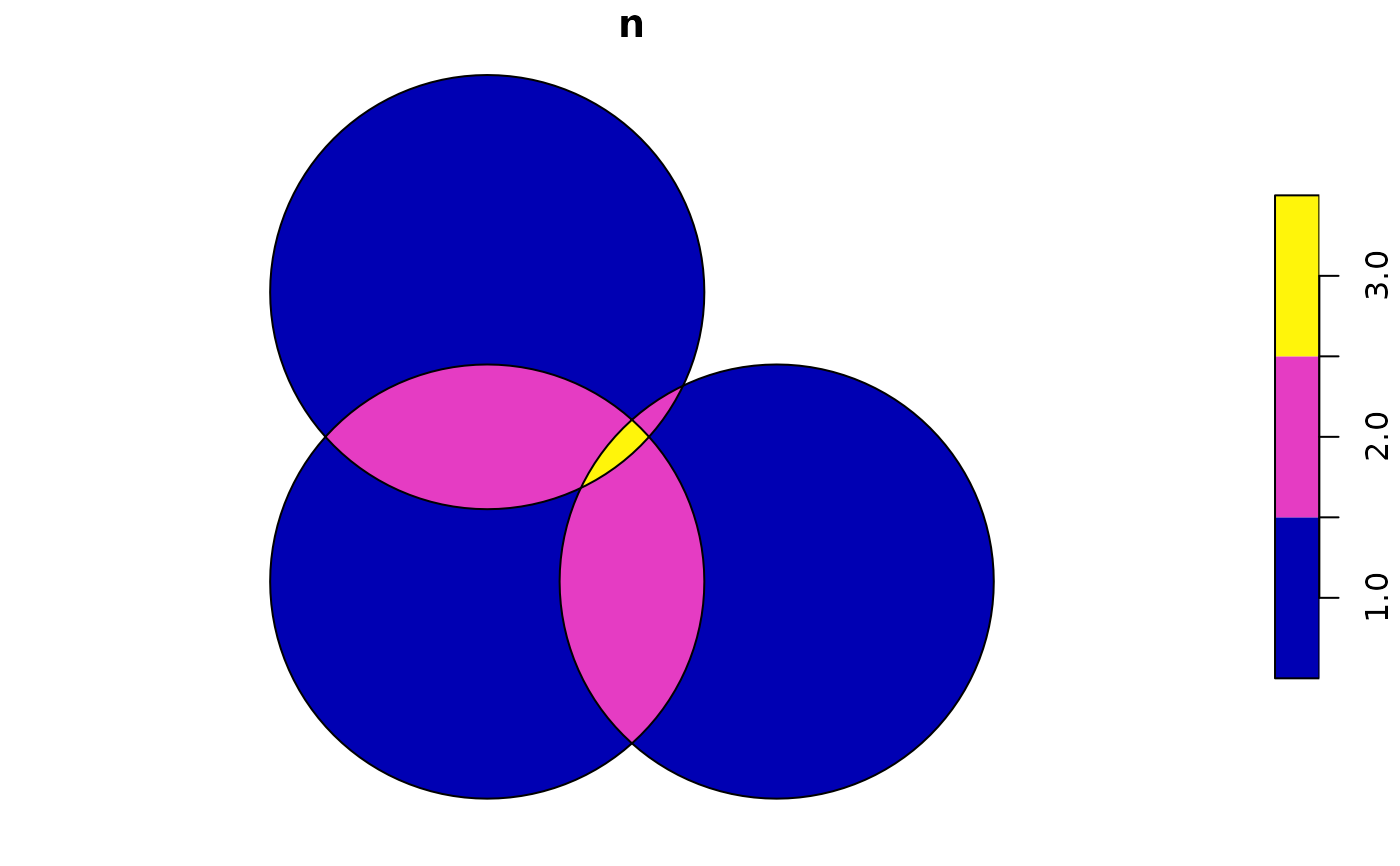Planar partition from disparate polygon inputs. Overlaps aggregate to `n`.

`ms_aggregate_polys(px, ...)`

## Arguments

px input polygons (assumed overlapping poly/mpolys) unused

## Details

Input is a single simple features polygon data frame. No attribute data is considered.

## Examples

```g <- sf::st_sfc(list(sf::st_point(cbind(0, 0)),
sf::st_point(cbind(0, 1)),
sf::st_point(cbind(1, 0))))
pts <- sf::st_sf(a = 1:3,  geometry = g)
overlapping_polys <- sf::st_buffer(pts, 0.75)

## decompose and count space-filling from overlapping polygons
x <- ms_aggregate_polys(overlapping_polys)# library(ggplot2)